# Conditional statements

Contents

### IF function basics

Conditional statements, conditional expressions, conditional processing, IF-THEN, or IF-THEN-ELSE are all names for a programming concept where conditions are tested and actions are performed if conditions are met. If a certain condition is met, something is calculated. If that certain condition is not met, either nothing happens or something else is calculated.

In Excel, when conditions are met, something is TRUE.

When conditions are not met, something is FALSE.

TRUE and FALSE are logical values. You will notice that Excel centers them if you return them to unformatted cells. They are not text, which is aligned left, and they are not numbers, which are aligned right.

The IF function will check IF something is TRUE, and THEN, if it is TRUE, perform a calculation. ELSE, when something is FALSE, a different calculation will be performed.

The syntax of the IF function is as follows:

= IF ( logical_test; value_if_true; value_if_false )

A logical test will always result in a logical value TRUE or a logical value FALSE.

Value if true can be either a number, a text string, another formula, or a function.

Value if false can also be either a number, a text string, another formula, or a function.

This is how the basic application of the IF function looks: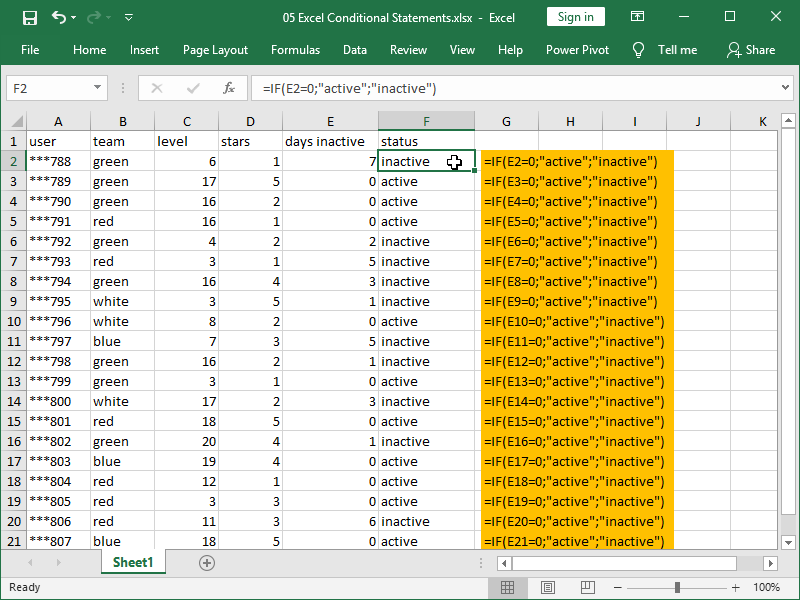The logical test reads as follows: if the number in column E is zero, then the statement is TRUE, else the statement is FALSE.

If TRUE then text “active” will be returned, else text “inactive” will be returned.

If we don’t specify what will be returned for either TRUE or FALSE, the IF function will return zero.

However, if we don’t want zero returned, we can return an empty text field using empty quotation marks “”: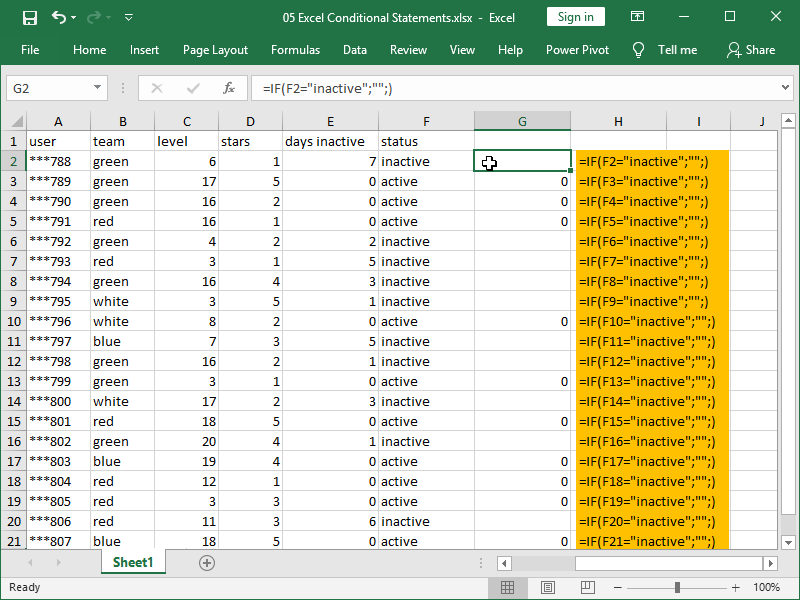The logical test reads as follows: if the text in the column E is the same as the text “inactive”, then the statement is TRUE, else the statement is FALSE.

If TRUE, then the text “” will be returned; as else is not defined, zero will be returned.

Note that the IF function is not case-sensitive; we would get the same results with the text “Inactive”.

Finally, we’ve already shown that you can perform logical tests with both numbers and text.

While writing your statements, make sure you are comparing two of the kind types of values – rules from previous lessons apply here.

1=1 is TRUE as both parts of the statements are numbers and the number in both cases is 1.

“1”=”1″ is TRUE as those two text strings are the same.

But 1=”1″ is FALSE as one part of the statement is a number and the other part is text, and they are thus not the same.

### Comparison operators

The simplest logical tests are performed with the help of comparison operators (also known as logical operators).

These are the Comparison operators available:

equal to =
greater than >
greater than or equal to >=
less than <
less than or equal to <=
not equal to <>

For example, we can use the less than or equal to operator <= to check which of our users are inactive (have not been active for 3 or more days):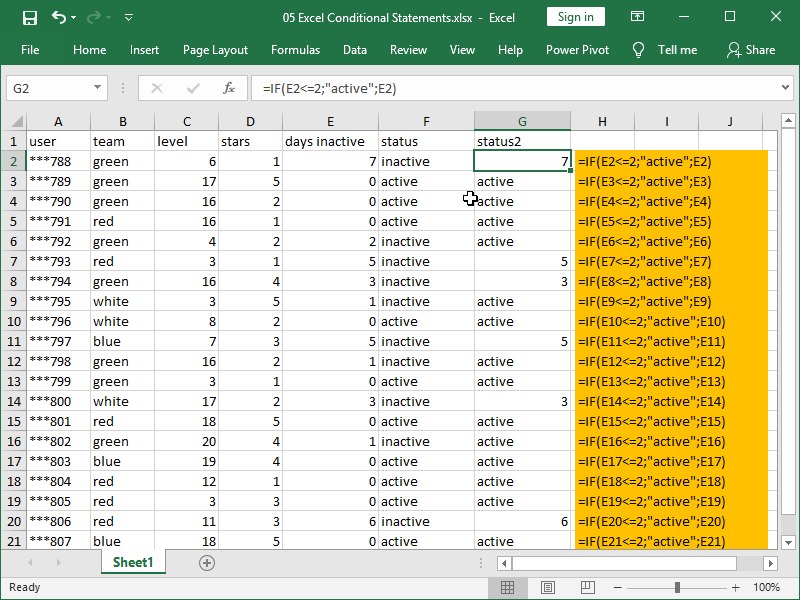The logical test reads as follows: if the number in column E is less than or equal to number 2, then the statement is TRUE, else the statement is FALSE.

If TRUE, then the text “active” will be returned, else the number of days inactive in column E will be returned.

Nothing is stopping us from using the greater than or equal to >= operator for the same purpose: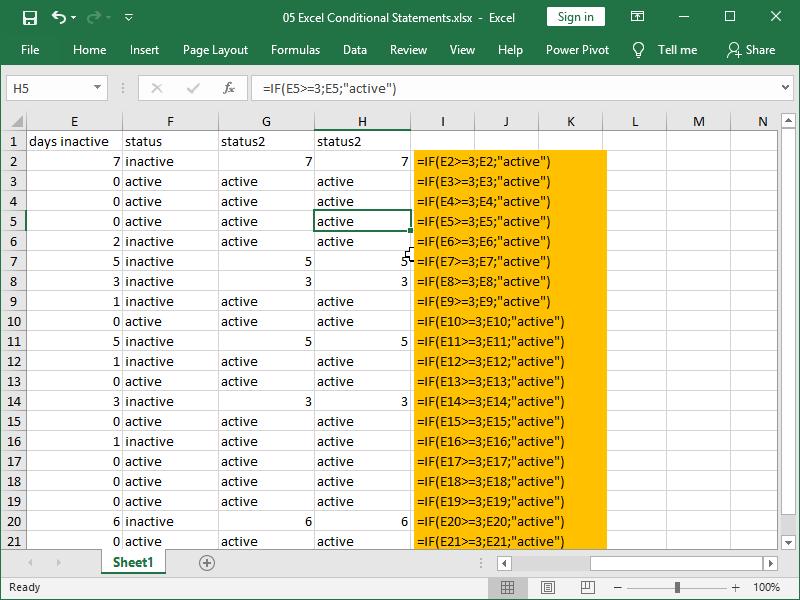The logical test reads as follows: if the number in column E is greater than or equal to number 3, then the statement is TRUE, else the statement is FALSE.

If TRUE then the number of days inactive in column E will be returned, else the text “active” will be returned.

### Nested IF statements

We can “nest” any general-purpose function inside of the IF function.

We can also “nest” one or more IF functions inside the IF function. This enables us to perform multiple different actions depending on the tested condition.

Suppose we want to give incentives (10 gold for each day of inactivity, with the current exchange rate of EUR 0,04517 for 10 gold) to our users who give us poor ratings (3 or less stars). We can calculate our incentive cost by nesting the ROUND function inside the IF function: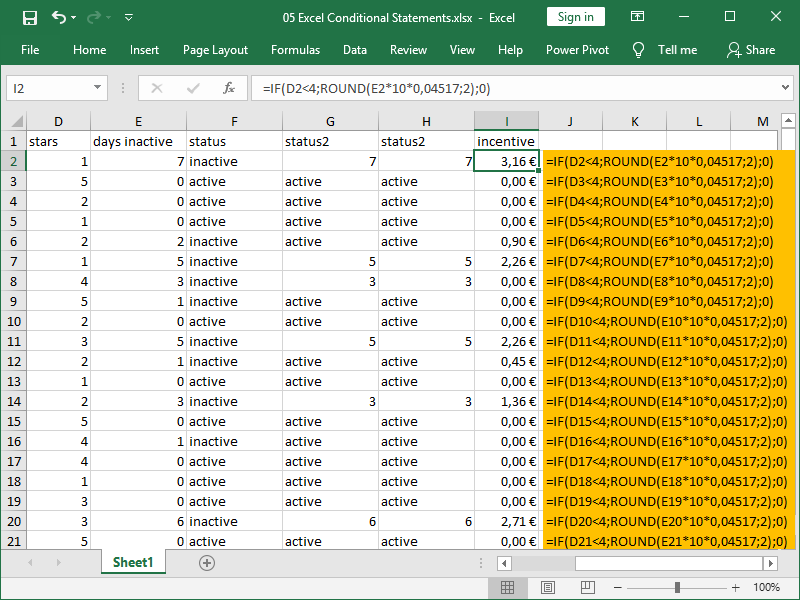The logical test reads as follows: if the number in column D is less than 4, then the statement is TRUE, else the statement is FALSE.

If TRUE then the number of inactive days will be multiplied by 10 (gold) and again multiplied by the gold exchange rate of 0,04517, rounded to two decimal points, and then returned, else zero will be returned.

Suppose we want to convert our number of stars into a descriptive rating, with 1 star being Abysmal, 2 Bad, 3 Passable, 4 Good, and 5 Excellent. We can accomplish that conversion by nesting multiple IF functions inside the IF function: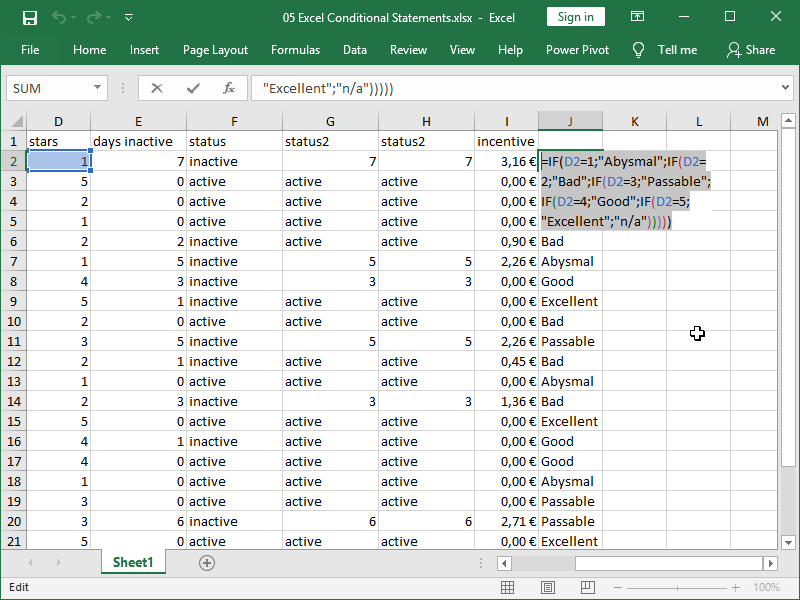In this example, every FALSE return starts a new logical test until all options are exhausted, and every FALSE statement except the last one contains a new IF function.

Confused yet? Don’t avoid sketching flowcharts representing your process first if they will help you construct your statements. This is how a flowchart for the function shown above would look: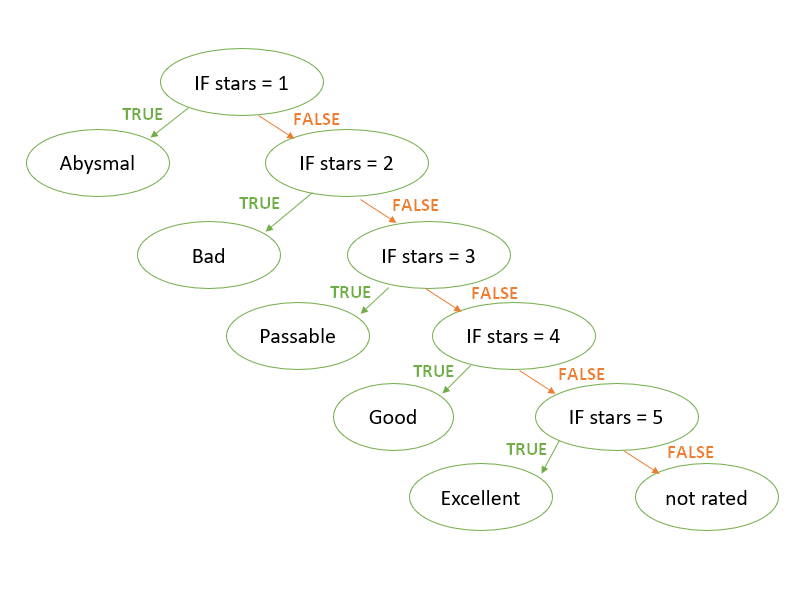The IFS function checks whether conditions are met and returns a value that corresponds to the first TRUE condition.

The syntax of the IFS function is as follows:

= IFS ( logical_test ; value_if_true ; logical_test ; value_if_true ; … )

However, with the IFS function, if no TRUE conditions are found, #N/A error is returned. You have to anticipate every possible condition while writing it in order to avoid errors.

### Simple logical tests

Other than performing logical tests using comparison operators, you can also return TRUE and FALSE with functions.

If you just want to return TRUE or FALSE, the easiest way is to use =TRUE() or =FALSE().

However, you will want to use functions to perform logical tests, with the end goal of “nesting” those functions inside an IF function.

The EXACT function compares whether two text strings are identical and returns TRUE or FALSE depending on the outcome.

The ISTEXT function checks whether the value is text or not and returns TRUE or FALSE depending on the outcome.

The ISNUMBER function checks whether the value is a number or not and returns TRUE or FALSE depending on the outcome.

The ISLOGICAL function checks whether the value is a logical value (TRUE or FALSE) or not and returns TRUE or FALSE depending on the outcome.

The ISBLANK function checks whether the cell is empty or not and returns TRUE or FALSE depending on the outcome.

The ISERROR function checks whether the cell or formula return is an error (#N/A, #VALUE!, #REF!, #DIV/0!, #NUM!, #NAME?, or #NULL!), and returns TRUE or FALSE depending on the outcome.

The ISERR function checks whether the cell or formula return is an error but ignores the #N/A error and returns TRUE or FALSE depending on the outcome.

The ISNA function checks whether the cell or formula returns the #N/A error and returns TRUE or FALSE depending on the outcome.

We will now test the figures in column E with these logical functions. We are expecting text, but there are many empty cells and data that Excel recognized as something other than text at import: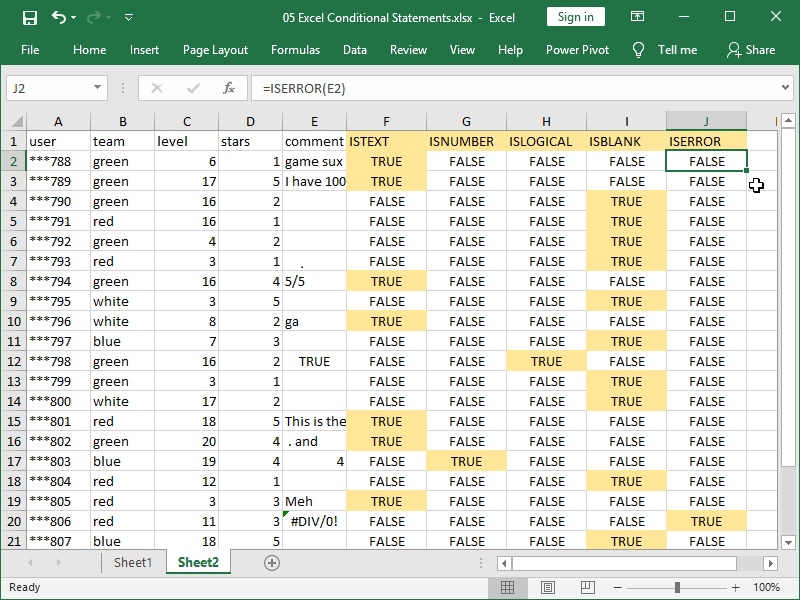We will now “nest” the ISERROR function inside of the IF function: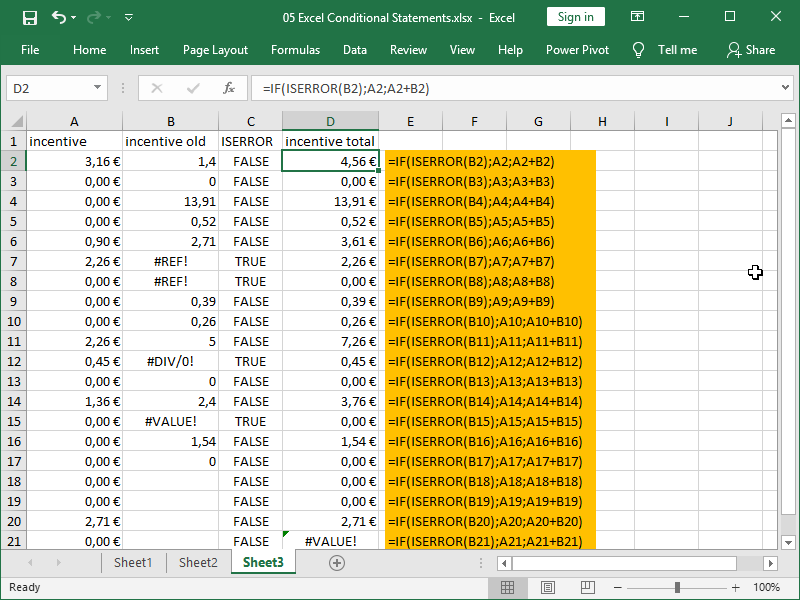The goal here is to perform the addition of columns A and B, but given the many errors in column B, we first have to check for those errors.

If the function returns TRUE, i.e., there is an error in column B, we will only take the number from column A.

If the function returns FALSE, i.e., there is no error in column B, we will sum columns A and B.

Note the issue with cell D21. Given that cell B21 contains a space character, the sum of cells A21 and B21 results in a new error.

One workaround we can use is to check whether the sum of columns A and B results in an error: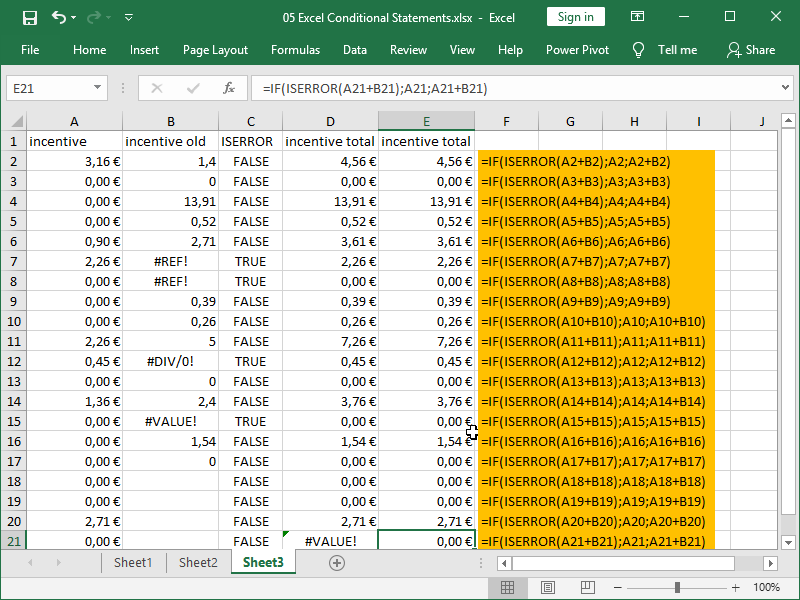Here, we first check if sum of column A and column B returns an error.

If the function returns TRUE, i.e. instead of sum we receive an error, we will only take number from column A.

If the function returns FALSE, there is no error in column B, and we will sum columns A and B.

Alternatively, checking for errors can also be performed with specialized functions using simpler syntax, i.e., the IFERROR function and the IFNA function.

### Complex logical tests

Sometimes multiple conditions need to be met for a certain calculation to be performed.

Rather than using complex nesting of multiple IF functions inside the IF function, we can perform our logical tests using specialized AND and OR functions.

The AND function tests multiple conditions and returns TRUE if all tested conditions are TRUE.

Suppose we want to identify users that are at level 10 or less, AND rate our application as three stars or less, AND have never received any incentive: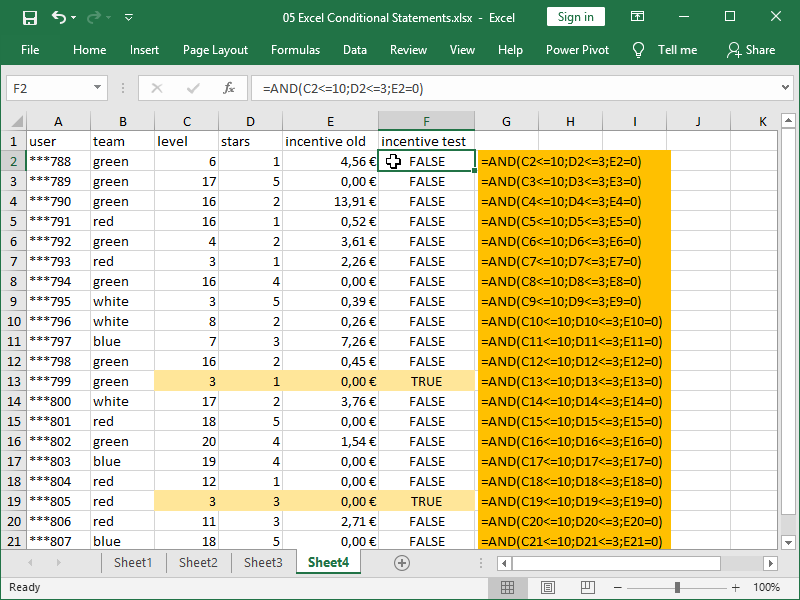The logical test reads as follows: if the number in column C is less than or equal to 10, AND the number in column D is less than or equal to 3, AND the number in column E is equal to zero, then the statement is TRUE, else the statement is FALSE.

Understanding this logic, we can “nest” this function inside the logical test part of the IF function: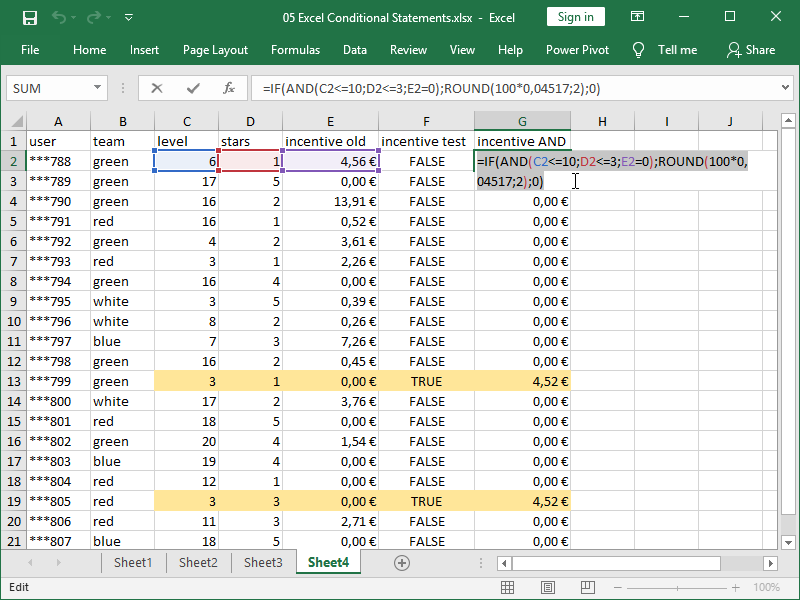If TRUE then 100 gold will be added to the user, else zero will be returned.

The OR function tests multiple conditions and returns TRUE if one of the tested conditions is TRUE.

Suppose we want to identify users that are at level 10 or less, OR rate our application as three stars or less, OR have never received any incentive: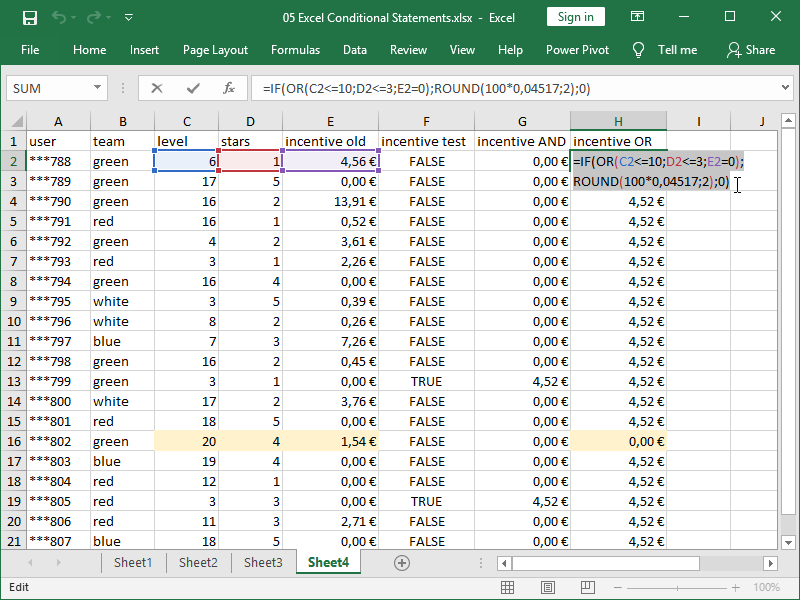The logical test reads as follows: if the number in column C is less than or equal to 10, OR the number in column D is less than or equal to 3, OR the number in column E is equal to zero, then the statement is TRUE, else the statement is FALSE.

If TRUE then 100 gold will be added to the user, else zero will be returned.

Note the difference: while there are only two users that meet all of the criteria, there is only one user that doesn’t meet any of the criteria.

Functions performing logical tests can also be nested one inside another.

Consider the following example: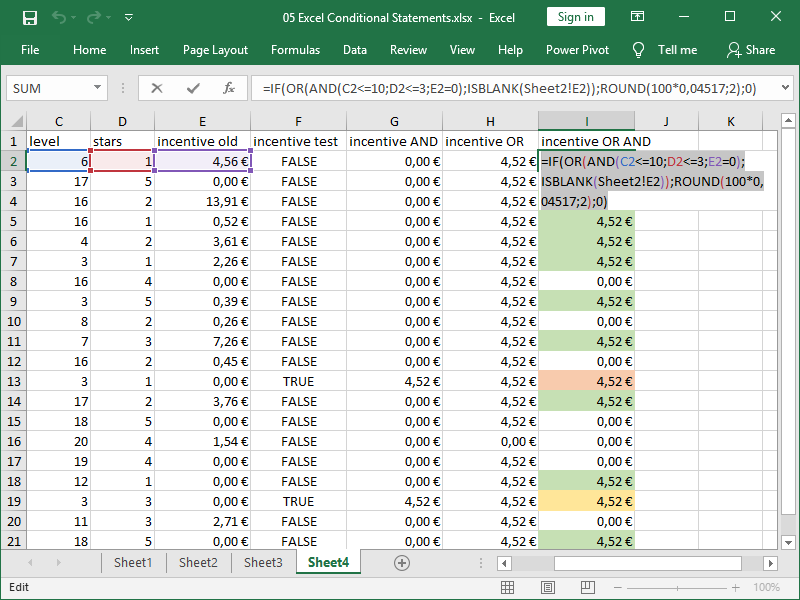We are first identifying users that are at level 10 or less, rate our application as three stars or less, and have never received any incentive (yellow, red).

If one is true or another is true, or both are true, we are incentivizing them.

Worth mentioning are also:

The XOR function, or exclusive OR function, returns TRUE when individual returns differ (TRUE;FALSE), and FALSE when individual returns are the same (TRUE;TRUE or FALSE;FALSE).

The NOT function returns FALSE when something is TRUE, and TRUE when something is FALSE. I.e., =NOT(FALSE()) returns TRUE and =NOT(TRUE()) returns FALSE.